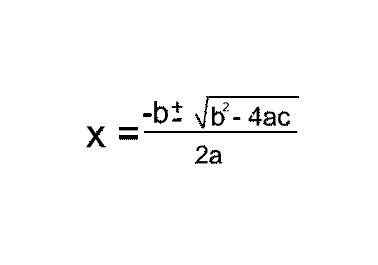# Basic kinematics problem

gldnsrfbord
Hey there. I've got a physics problem that is troubling me. I feel like I should be able to do it, but i's been a while since I've done much physics and I could use some help.

## Homework Statement

Mike jumps off a springy diving board located 10.0m above the surface of a swimming pool on the moon. He launches straight up at 15.0 m/s. (assume he misses the diving board on the way back down.) If gmoon is exactly one-sixth gearth, at what time does he hit the water?

## Homework Equations

I used, x=x0+v0t+1/2at2, but my answers didn't work when I plugged them back in, I think my math may be way off or something.

## The Attempt at a Solution

0=10+(15)t+(1/2)(-9.80/6)t2
0=10+15t-(9.80/12)t2
-10=t(15-(9.80/12)t)

t= -10, 30.61

It can't be -10, but when I plug both of them back in to the original equation, it never works out.
Thanks in advance for any help!
-Tom

Homework Helper
Gold Member
Hey there. I've got a physics problem that is troubling me. I feel like I should be able to do it, but i's been a while since I've done much physics and I could use some help.

## Homework Statement

Mike jumps off a springy diving board located 10.0m above the surface of a swimming pool on the moon. He launches straight up at 15.0 m/s. (assume he misses the diving board on the way back down.) If gmoon is exactly one-sixth gearth, at what time does he hit the water?

## Homework Equations

I used, x=x0+v0t+1/2at2, but my answers didn't work when I plugged them back in, I think my math may be way off or something.

## The Attempt at a Solution

0=10+(15)t+(1/2)(-9.80/6)t2
0=10+15t-(9.80/12)t2
yes, solve for t using the quadratic formula solution t = -b +/ sq rt....etc.
-10=t(15-(9.80/12)t)

t= -10, 30.61
no, math is off.
It can't be -10, but when I plug both of them back in to the original equation, it never works out.
Thanks in advance for any help!
-Tom

Last edited:
Mentor
Hey there. I've got a physics problem that is troubling me. I feel like I should be able to do it, but i's been a while since I've done much physics and I could use some help.

## Homework Statement

Mike jumps off a springy diving board located 10.0m above the surface of a swimming pool on the moon. He launches straight up at 15.0 m/s. (assume he misses the diving board on the way back down.) If gmoon is exactly one-sixth gearth, at what time does he hit the water?

## Homework Equations

I used, x=x0+v0t+1/2at2, but my answers didn't work when I plugged them back in, I think my math may be way off or something.

## The Attempt at a Solution

0=10+(15)t+(1/2)(-9.80/6)t2
0=10+15t-(9.80/12)t2
-10=t(15-(9.80/12)t)

t= -10, 30.61

It can't be -10, but when I plug both of them back in to the original equation, it never works out.
Thanks in advance for any help!
-Tom

Your initial equation looks correct. Try solving it with the Quadratic Formula:Homework Helper
When ab = 0, then it is true that a = 0 or b = 0. But when ab = -10, it is not true that a = -10 or b = -10.

That logic can only be used when there is no constant factor. You need to complete the square or use the quadratic formula to find the values of t. The formula is much easier to understand and remember if you know how to complete the square.

Mentor
Holy cow! We triple-teamed!

gldnsrfbord
Thanks guys, I guys a couple years away from algebra lead to me completely forgetting how to do it!

I'm plugging (-15+sqroot(152+(98.0/3)))/(9.80/6) and getting ~0.644. Does that sound right? I'm really not too sure about that. And when I change the + to a - I get -19.011. Is there still something that I'm forgetting to do?

Mentor
Thanks guys, I guys a couple years away from algebra lead to me completely forgetting how to do it!

I'm plugging (-15+sqroot(152+(98.0/3)))/(9.80/6) and getting ~0.644. Does that sound right? I'm really not too sure about that. And when I change the + to a - I get -19.011. Is there still something that I'm forgetting to do?

Doesn't look quite right. First of all, there is no 2x in the denominator. And the 2nd term inside the radical doesn't look right either. I could just be having trouble reading the equation without Latex, though.